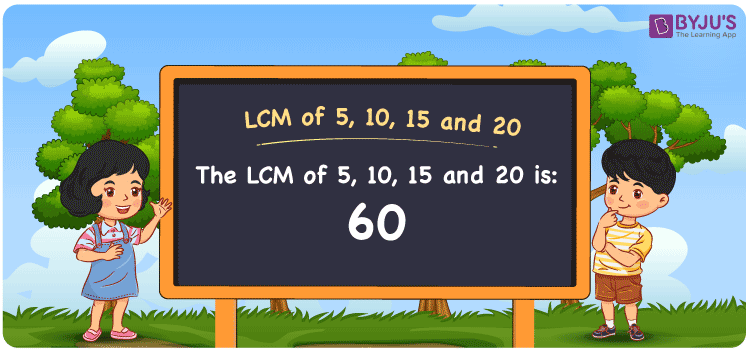Checkout JEE MAINS 2022 Question Paper Analysis : Checkout JEE MAINS 2022 Question Paper Analysis :

# LCM of 5, 10, 15 and 20

LCM of 5, 10, 15 and 20 is 60. The LCM is a very important concept in Mathematics and has a wide application in our daily lives. Least common multiple of 5, 10, 15 and 20 is the smallest number which we get from the common multiples. (5, 10, 15, 20, 25, ….), (10, 20, 30, 40, 50,….), (15, 30, 45, 60, 75, …..) and (20, 40, 60, 80, 100, …..) are the first few multiples of 5, 10, 15 and 20. The LCM can be found by using methods such as division, prime factorisation and by listing the multiples.

## What is LCM of 5, 10, 15 and 20?

The answer to this question is 60. The LCM of 5, 10, 15 and 20 using various methods is shown in this article for your reference. The LCM of two non-zero integers, 5, 10, 15 and 20, is the smallest positive integer 60 which is divisible by both 5, 10, 15 and 20 with no remainder.## How to Find LCM of 5, 10, 15 and 20?

LCM of 5, 10, 15 and 20 can be found using three methods:

• Prime Factorisation
• Division method
• Listing the multiples

### LCM of 5, 10, 15 and 20 Using Prime Factorisation Method

The prime factorisation of 5, 10, 15 and 20, respectively, is given by:

5 = 5¹

10 = 2 x 5 = 2¹ x 5¹

15 = 3 x 5 = 3¹ x 5¹

20 = 2 x 2 x 5 = 2² x 5¹

LCM (5, 10, 15, 20) = 60

### LCM of 5, 10, 15 and 20 Using Division Method

We’ll divide the numbers (5, 10, 15, 20) by their prime factors to get the LCM of 5, 10, 15 and 20 using the division method (preferably common). The LCM of 5, 10, 15 and 20 is calculated by multiplying these divisors.

 2 5 10 15 20 3 5 5 15 10 5 5 5 5 10 2 1 1 1 2 x 1 1 1 1

No further division can be done.

Hence, LCM (5, 10, 15, 20) = 60

### LCM of 5, 10, 15 and 20 Using Listing the Multiples

To calculate the LCM of 5, 10, 15 and 20 by listing out the common multiples, list the multiples as shown below

 Multiples of 5 Multiples of 10 Multiples of 15 Multiples of 20 5 10 15 20 10 20 30 40 15 30 45 60 20 40 60 80 25 50 75 100 30 60 90 120 35 70 105 140 40 80 120 160 45 90 135 180 50 100 150 200 55 110 165 220 60 120 180 240

The smallest common multiple of 5, 10, 15 and 20 is 60.

Therefore LCM (5, 10, 15, 20) = 60

## Video Lesson on Applications of LCM## LCM of 5, 10, 15 and 20 Solved Examples

Question: Calculate the smallest number which is divisible exactly by 5, 10, 15 and 20.

Solution:

The smallest number which is exactly divisible by 5, 10, 15 and 20 is the LCM.

Multiples of 5 = 5, 10, 15, 20, 25, 30, 35, 40, 45, 50, 55, 60, …..

Multiples of 10 = 10, 20, 30, 40, 50, 60, ….

Multiples of 15 = 15, 30, 45, 60, 75, ….

Multiples of 20 = 20, 40, 60, 80, 100, ….

Hence, the LCM is 60.

## Frequently Asked Questions on LCM of 5, 10, 15 and 20

### Using the prime factorisation, find the LCM of 5, 10, 15 and 20.

The LCM of 5, 10, 15 and 20 is 60.

Using the prime factorisation

5 = 5¹

10 = 2 x 5 = 2¹ x 5¹

15 = 3 x 5 = 3¹ x 5¹

20 = 2 x 2 x 5 = 2² x 5¹

LCM (5, 10, 15, 20) = 60

### Which methods can be used to find the LCM of 5, 10, 15 and 20?

The methods which can be used to find the LCM of 5, 10, 15 and 20 are Prime Factorization Method, Division Method and Listing multiples.

### Among 110, 42, 35, 60, find the LCM of 5, 10, 15 and 20.

The LCM of 5, 10, 15 and 20 is the smallest common multiple which is divisible exactly by the given numbers. The number which satisfies this condition is 60.